Get instant live expert help with Excel or Google Sheets“My Excelchat expert helped me in less than 20 minutes, saving me what would have been 5 hours of work!”

#### Post your problem and you'll get expert help in seconds

Your message must be at least 40 characters
Our professional experts are available now. Your privacy is guaranteed.

# Sum by group

While working with Excel, we are able to sum values that satisfy more than one criteria by using the SUMIF function.  This step by step tutorial will assist all levels of Excel users in summing values by group using the IF and SUMIF functions.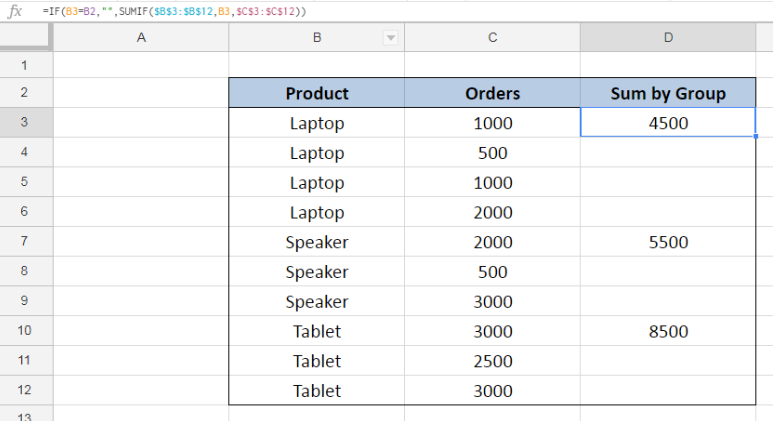Figure 1. Final result: Sum by group

Final formula: `=IF(B3=B2,"",SUMIF(\$B\$3:\$B\$12,B3,\$C\$3:\$C\$12))`

## Syntax of IF Function

IF function evaluates a given logical test and returns a TRUE or a FALSE

`=IF(logical_test, [value_if_true], [value_if_false])`

• The arguments “value_if_true” and “value_if_false” are optional.  If left blank, the function will return TRUE if the logical test is met, and FALSE if otherwise.

## Syntax of the SUMIF Function

SUMIF sums the values in a specified range, based on one given criteria

`=SUMIF(range,criteria, [sum_range])`

The parameters are:

• Range: the data range that will be evaluated using the criteria
• Criteria: the criteria or condition that determines which cells will be added
• Sum_range: the cells that will be added; if left blank, “sum_range” = “range” which means that the range of data that will be added is the same range of data evaluated

## Setting up the Data

Our table has three columns: Product (column B), Orders (column C) and Sum by Group (column D).  We want to group the products and calculate the sum by group. The results will be recorded in column D.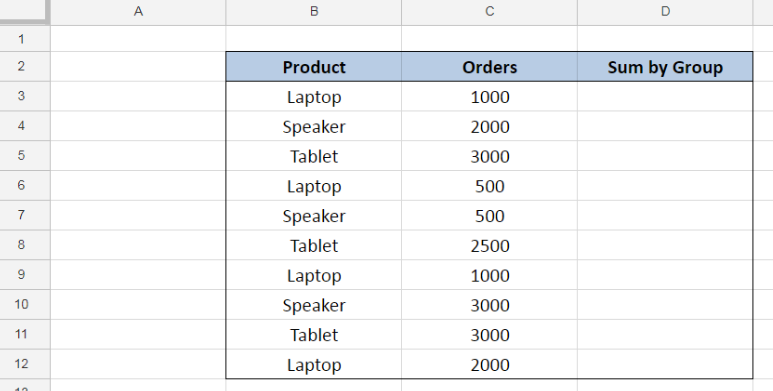Figure 2. Sample data to sum by group

We have three products namely: Laptop, Speaker and Tablet. First, we need to sort our data by product through these steps:

Step 1.  Select the range for our data, B2:D12

Step 2.  Click the Data tab, then select Create a filter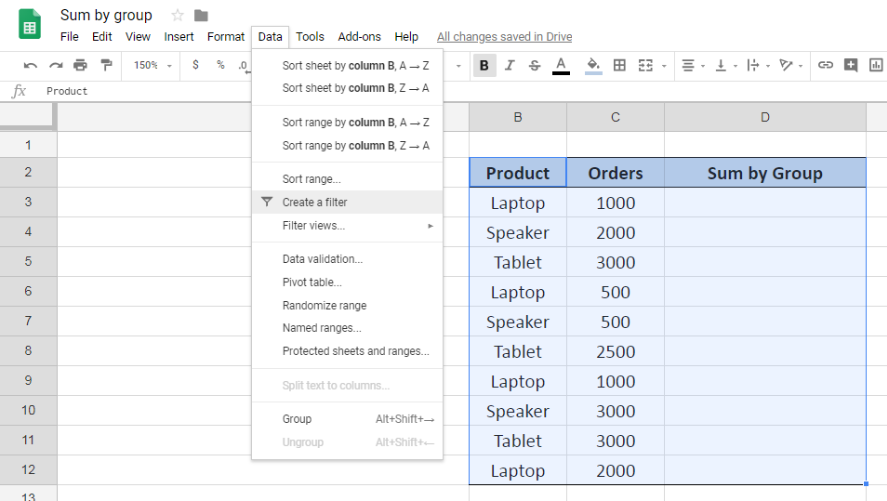Figure 3. Creating a filter to sort data by product

Step 3.  Click the drop-down arrow for Product

Step 4.  Select Sort A-Z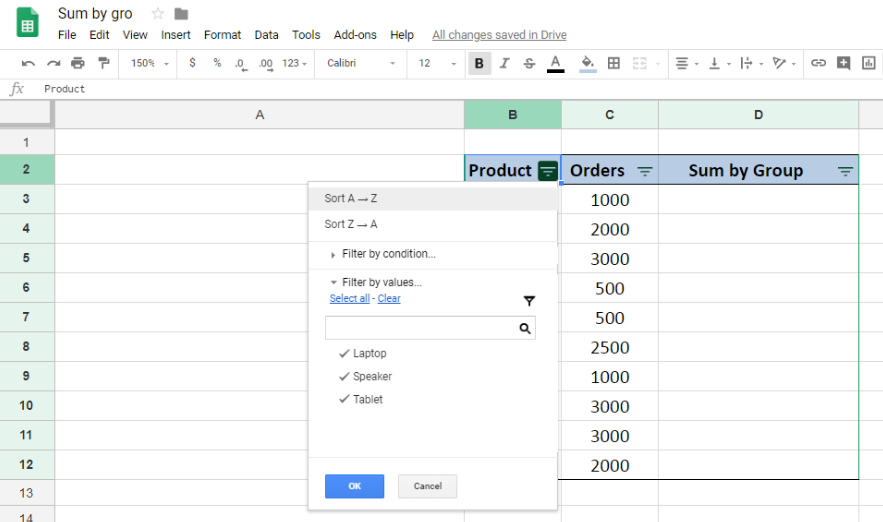Figure 4. Sorting the data by product

The resulting table will show the products arranged alphabetically.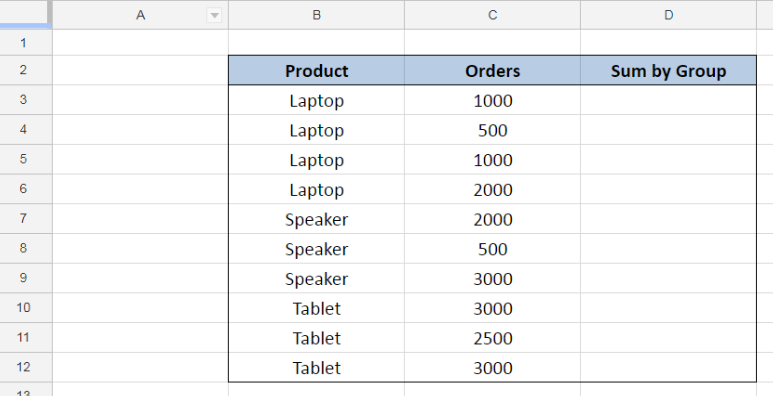Figure 5. Output: Sorting the data by product through Excel filter

## Sum orders by product

Now that we have sorted our data by product, we can now sum the orders per product by using the IF and SUMIF functions.  Our goal is to show the sum on the first row that a product appears. Let us follow these steps:

Step 1.  Select cell D3

Step 2.  Enter the formula: `=IF(B3=B2,"",SUMIF(\$B\$3:\$B\$12,B3,\$C\$3:\$C\$12))`

Step 3.  Press Enter

Step 4:  Copy the formula in cell D3 to cells D4:D12 by clicking the “+” icon at the bottom-right corner of cell D3 and dragging it down

Our formula evaluates if the value in B3 is equal to the value of the previous cell B2.  If the logical test returns TRUE, the IF function will return an empty string ””. Otherwise, it will sum the orders in column C corresponding to the product in column B.

Since B3 “Laptop” is not equal to B2 “Product”, our formula returns the sum of orders for “Laptop”.  As a result, the total orders for Laptop in cell D3 is 4500, which is the sum of 1000, 500, 1000 and 2000 in cells C3:C6.

For cells D4:D6, our formula returns as empty string “” because the value in B4:B6 is equal to the cell before it, which is Laptop.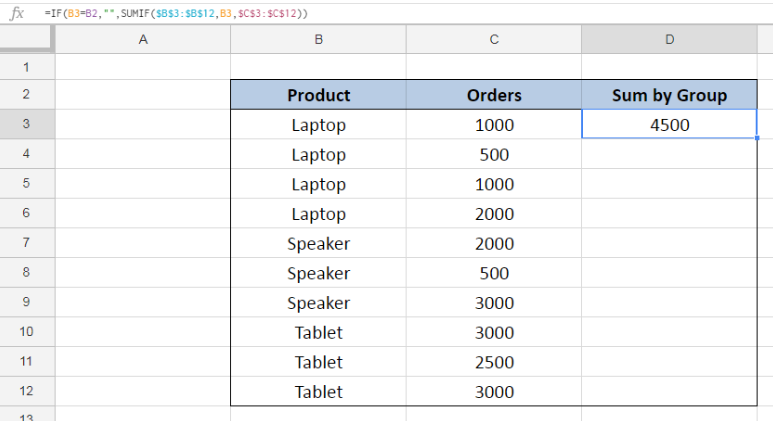Figure 6. Entering the formula using IF and SUMIF to sum orders by product

For the succeeding cells in column D, our formula returns the sum of orders for “Speaker” and “Tablet” in cell D7 and D10, respectively.  The cells in between are blank or empty because those are evaluated TRUE by our IF formula.

Below table shows the final result for the sum of orders per product.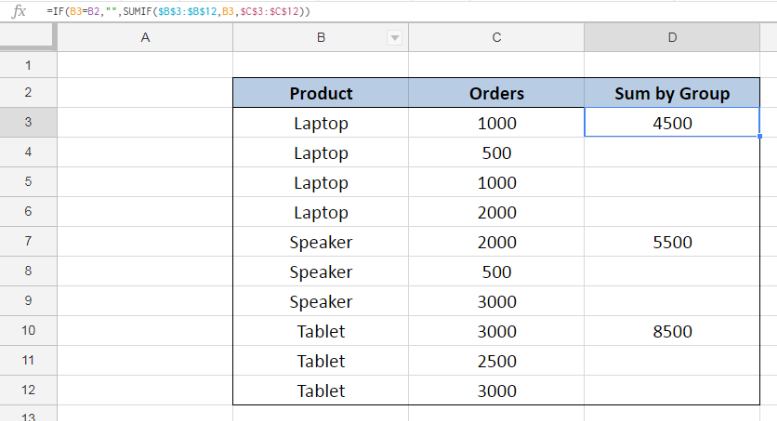Figure 7.  Output: Using IF and SUMIF to sum orders by product

Most of the time, the problem you will need to solve will be more complex than a simple application of a formula or function. If you want to save hours of research and frustration, try our live Excelchat service! Our Excel Experts are available 24/7 to answer any Excel question you may have. We guarantee a connection within 30 seconds and a customized solution within 20 minutes.

### Did this post not answer your question? Get a solution from connecting with the expert.Another blog reader asked this question today on Excelchat:## Subscribe to Excelchat.coAnother blog reader asked this question today on Excelchat: